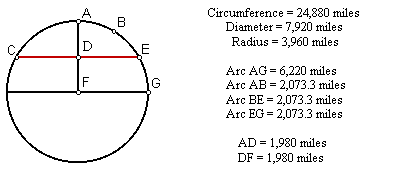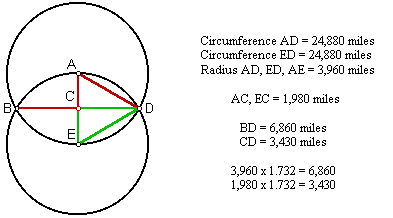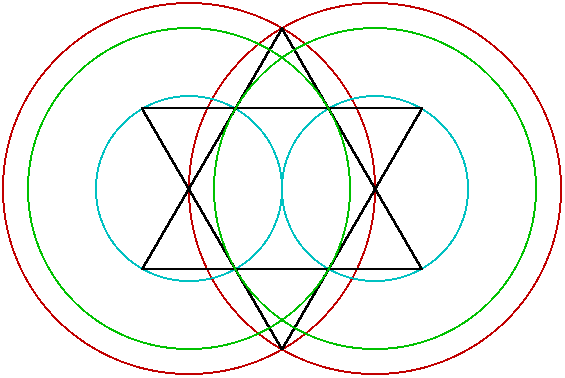THE 30TH PARALLEL

The horizontal axis of this spherical earth model represents the equator, CE is the 30th parallel, B is at 60° north latitude and A is the North Pole. The 30th parallel is one-third of the great circle distance from the equator to the North Pole, and it is located at one-half of the height of the northern hemisphere. Like the Great Pyramid, the maximum latitude of the line of ancient sites is very close to the 30th parallel.This diagram below illustrates that the relationship of the 30th parallel to the circumference of a spherical earth model is the geometric relationship known as the Vesica Pisces. In relation to the lower circumference, BD is at 30° N latitude. In relation to the upper circumference, BD is at 30° S latitude. The ratio between the straight line distance of the 30th parallel and the radius of the earth is 1.732 to one, an exact expression of the square root of three.

In relation to the lower circumference, A represents the North Pole. Both circles are the same size and D is on the circumference of both circles, demonstrating that sites on the 30th parallel, such as the Great Pyramid, are equally distant from the North Pole and the center of the earth.ADE is an equilateral triangle with each side equal to the radius of this spherical earth model. CD bisects ADE, forming two 90° 60° 30° right triangles. The short side of a 90° 60° 30° right triangle (CE) is exactly one half of the length of the hypotenuse (ED) and the long side (CD) is equal to the short side times the square root of three:

1 ˛ + √3 ˛ = 2 ˛

The diagramed earth measures for CE, CD and ED produce the same result:

1980 ˛ + 3430 ˛ = 3960 ˛

BD is twice the length of CD. The measures of the inscribed 90° 60° 30° right triangle demonstrate that the straight line distance of the 30th parallel (BD) is equal to the radius of the earth times the square root of three:

3960 miles x 1.732 = 6860 miles

The Vesica Pisces describes the square root of three and equilateral triangles describe the square root of three over two. The hexagram is comprised of equilateral triangles and the diagram below shows the correspondences between a Vesica Pisces and a hexagram. All of the circles are centered on the two intersection points at the middle height of the hexagram. The red circles have a radius of two inches and form a Vesica Pisces.The blue circles have a radius of one inch. The green circles have a radius of 1.732 inches. The distance between the two intersection points at the middle height of the hexagram is two inches. The length of the sides of the two large equilateral triangles is three inches. The length of the sides of the six small equilateral triangles is one inch, equal to the radius of the blue circles.

The points where the green circles touch the intersection points of the hexagram are 1.732 inches away from the points where the green circles are centered on the intersection points at the middle height of the hexagram.

Unlike the diagrams above, the earth is not perfectly spherical. It is slightly wider at the equator and slightly flattened at the poles. This is primarily due to the centrifugal force generated by the spin of the earth on it's axis. As a result, the curvature of the earth is greater towards the equator, and less towards the poles, causing the length of degrees of latitude to increase very slightly from the equator to the poles. The length of one degree of latitude from the equator to 1° north is 68.71 miles. The length of one degree of latitude from 89° north to the north pole is 69.4 miles. 68.71 miles is equal to 362776 feet, divided by sixty equals 6046 feet per minute of latitude, divided by two equals 3023 feet for one-half minute of latitude at the equator. This is the precise length of the perimeter of the Great Pyramid.

With baselengths of 440 cubits per side, the perimeter of the Great Pyramid is 1,760 cubits long. The ancient Egyptian cubit equals one and a half ancient Egyptian feet. The 1,760 cubit perimeter of the Great Pyramid is 2,640 ancient Egyptian feet long. Since this distance equals one half of one minute of latitude at the equator, 5,280 ancient Egyptian feet (the same number of feet found in the English mile) equals the length of one minute of latitude at the equator.

As shown by the Vesica Pisces diagrams, a degree of longitude at the 30th parallel is equal to a degree of longitude at the equator times the square root of three over two (.866):

69.17 miles x .866 = 59.9 miles

The most current Global Positioning Survey data shows that one degree of longitude is exactly 60 miles long at 29.92° north, in the immediate vicinity of Giza and the other pyramid fields just north of ancient Memphis. At this latitude, one minute of longitude is equal to one mile.

A number of additional correspondences exist between the measures of the Great Pyramid in cubits and measures of the earth in miles. The equatorial circumference of the earth is 24,900 miles. The perimeter of the Great Pyramid is 1,760 cubits. 1,760 times the square root of two (1.4142) equals 2,490. The mean radius of the earth is 3,960 miles. The height of the Great Pyramid is 280 cubits. 280 times the square root of two equals 396. One half of the radius of the earth is 1,980 miles. The base lengths of the Great Pyramid at the height that the upper shafts exit the pyramid are 198 cubits.

The values in modern English feet for the ancient Roman and Greek feet are as follows:

Roman: .9732096

Greek: 1.01376

In The New View Over Atlantis John Michell points out that the ratio between the lengths of these two measures is the simple fraction of 24/25:

.9732096/1.01376 = 24/25

Since both Roman and Greek miles were comprised of 5000 feet, 25 Roman miles also equals 24 Greek miles:

.9732096 x 5000 = 4866.048 feet

1.01376 x 5000 = 5068.8 feet

4866.048/5068.8 = 24/25

There are 5280 feet in an English mile. Michell also noted that 25 Greek miles equals 24 English miles:

5068.8/5280 = 24/25

The length of the ancient Sumerian foot and the ancient Saxon foot were both equal to 1.1 English feet. The ancient Egyptian cubit of 20.625 inches gives an ancient Egyptian foot of 13.75 inches or 1.1458 English feet. John Neal pointed out that the ratio between the length of the ancient Sumerian foot and the ancient Egyptian foot is also 24/25:

1.1/1/1.458 = 24/25

Assuming 5000 feet in both the Sumerian and the ancient Egyptian miles, 25 Sumerian miles also equals 24 ancient Egyptian miles:

1.1 feet x 5000 = 5500 English feet

1.1458 feet x 5000 = 5729 English feet

5500/5729 = 24/25

The ratio between the English mile of 5,280 feet and the Sumerian/Saxon mile of 5,500 English feet is also the fraction of 24/25:

5280/5500 = 24/25

Thus, the relationships between all five of these measures are precisely defined by the fraction of 24/25:

25 Roman miles equals 24 Greek miles:

4866.048/5068.8 = 24/25

25 Greek miles equals 24 English miles:

5068.8/5280 = 24/25

25 English miles equals 24 Sumerian miles:

5280/5500 = 24/25

25 Sumerian miles equals 24 ancient Egyptian miles:

5500/5729 = 24/25